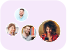Product Edition:1st Edition
Author: Ming-Seng Kao
Book Name: Understanding Electromagnetic Waves
Subject Name: Engineering

# Understanding Electromagnetic Waves 1st Edition Solutions

0 out of 5.0
30 reviews31 Students
have requested for homework help from this book

This one-semester textbook teaches students Electromagnetic Waves, via an early introduction to Maxwellâ€™s Equations in the first chapter. Mathematics fundamentals are used as needed, but rigor is de-emphasized in preference to understanding the basic ideas and principles of EM waves. Each chapter includes extensive, step-by-step, solved examples, as well as abundant exercises. _x000D_ _x000D_ Designed for a one-semester course in electromagnetic waves;_x000D_ Introduces Maxwellâ€™s equations in the first chapter;_x000D_ De-emphasizes mathematical rigor in order to make key ideas and principles easy to understand;_x000D_ Makes material accessible to readers of varying backgrounds, with extensive use of solved examples;_x000D_ Includes abundant exercises for each chapter.

5
15
4
6
3
6
2
6
1
0

0

## Students who viewed this book also checked out